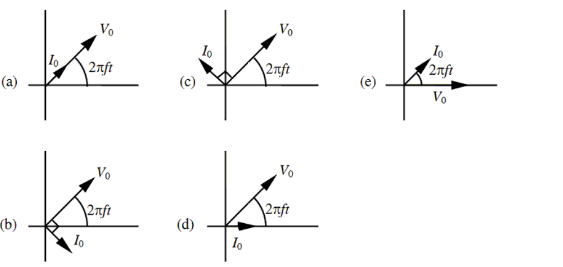Physics Practice Problems Phasors for Inductors Practice Problems Solution: Which one of the following phasor models correctly...

# Solution: Which one of the following phasor models correctly represents a circuit comprised of only an inductor and an ac generator?

###### Problem

Which one of the following phasor models correctly represents a circuit comprised of only an inductor and an ac generator?